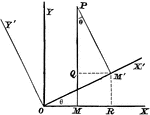### Rectangular Axes

Transforming from one set of rectangular axes to another with the same origin, but different direction.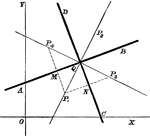### Line Bisectors

Multiple lines showing many bisectors on the coordinate plane.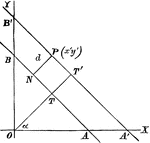### Perpendicular Distance

Finding the perpendicular distance of points whose equation is x cosa + y sina = p.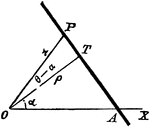### Polar Equation

Finding the polar equation of a straight line.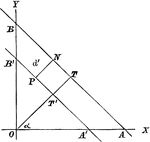### Alternate Form, Perpendicular Distance

An alternate form of the perpendicular distance method.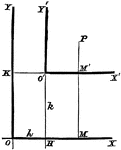### Axes Shift

Any system of coordinate axes to another set which is parallel to the former, but with different origins.